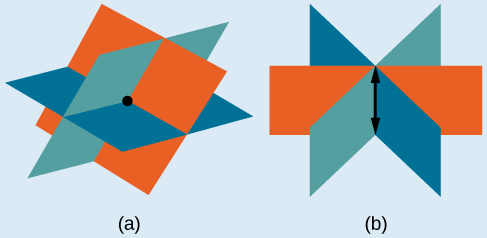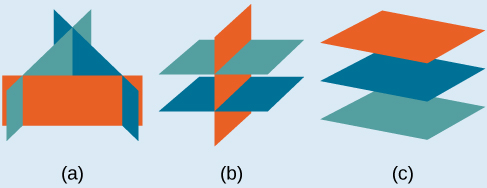# 11.2 Systems of linear equations: three variables

 Page 1 / 8
In this section, you will:
• Solve systems of three equations in three variables.
• Identify inconsistent systems of equations containing three variables.
• Express the solution of a system of dependent equations containing three variables.

John received an inheritance of $12,000 that he divided into three parts and invested in three ways: in a money-market fund paying 3% annual interest; in municipal bonds paying 4% annual interest; and in mutual funds paying 7% annual interest. John invested$4,000 more in municipal funds than in municipal bonds. He earned \$670 in interest the first year. How much did John invest in each type of fund?

Understanding the correct approach to setting up problems such as this one makes finding a solution a matter of following a pattern. We will solve this and similar problems involving three equations and three variables in this section. Doing so uses similar techniques as those used to solve systems of two equations in two variables. However, finding solutions to systems of three equations requires a bit more organization and a touch of visual gymnastics.

## Solving systems of three equations in three variables

In order to solve systems of equations in three variables, known as three-by-three systems, the primary tool we will be using is called Gaussian elimination , named after the prolific German mathematician Karl Friedrich Gauss . While there is no definitive order in which operations are to be performed, there are specific guidelines as to what type of moves can be made. We may number the equations to keep track of the steps we apply. The goal is to eliminate one variable at a time to achieve upper triangular form , the ideal form for a three-by-three system because it allows for straightforward back-substitution to find a solution $\text{\hspace{0.17em}}\left(x,y,z\right),\text{}$ which we call an ordered triple . A system in upper triangular form looks like the following:

The third equation can be solved for $\text{\hspace{0.17em}}z,\text{}$ and then we back-substitute to find $\text{\hspace{0.17em}}y\text{\hspace{0.17em}}$ and $\text{\hspace{0.17em}}x.\text{\hspace{0.17em}}$ To write the system in upper triangular form, we can perform the following operations:

1. Interchange the order of any two equations.
2. Multiply both sides of an equation by a nonzero constant.
3. Add a nonzero multiple of one equation to another equation.

The solution set to a three-by-three system is an ordered triple $\text{\hspace{0.17em}}\left\{\left(x,y,z\right)\right\}.\text{\hspace{0.17em}}$ Graphically, the ordered triple defines the point that is the intersection of three planes in space. You can visualize such an intersection by imagining any corner in a rectangular room. A corner is defined by three planes: two adjoining walls and the floor (or ceiling). Any point where two walls and the floor meet represents the intersection of three planes.

## Number of possible solutions

• Systems that have a single solution are those which, after elimination, result in a solution set    consisting of an ordered triple $\text{\hspace{0.17em}}\left\{\left(x,y,z\right)\right\}.\text{\hspace{0.17em}}$ Graphically, the ordered triple defines a point that is the intersection of three planes in space.
• Systems that have an infinite number of solutions are those which, after elimination, result in an expression that is always true, such as $\text{\hspace{0.17em}}0=0.\text{\hspace{0.17em}}$ Graphically, an infinite number of solutions represents a line or coincident plane that serves as the intersection of three planes in space.
• Systems that have no solution are those that, after elimination, result in a statement that is a contradiction, such as $\text{\hspace{0.17em}}3=0.\text{\hspace{0.17em}}$ Graphically, a system with no solution is represented by three planes with no point in common.(a)Three planes intersect at a single point, representing a three-by-three system with a single solution. (b) Three planes intersect in a line, representing a three-by-three system with infinite solutions.All three figures represent three-by-three systems with no solution. (a) The three planes intersect with each other, but not at a common point. (b) Two of the planes are parallel and intersect with the third plane, but not with each other. (c) All three planes are parallel, so there is no point of intersection.

what is the function of sine with respect of cosine , graphically
tangent bruh
Steve
cosx.cos2x.cos4x.cos8x
sinx sin2x is linearly dependent
what is a reciprocal
The reciprocal of a number is 1 divided by a number. eg the reciprocal of 10 is 1/10 which is 0.1
Shemmy
Reciprocal is a pair of numbers that, when multiplied together, equal to 1. Example; the reciprocal of 3 is ⅓, because 3 multiplied by ⅓ is equal to 1
Jeza
each term in a sequence below is five times the previous term what is the eighth term in the sequence
I don't understand how radicals works pls
How look for the general solution of a trig function
stock therom F=(x2+y2) i-2xy J jaha x=a y=o y=b
sinx sin2x is linearly dependent
cr
root under 3-root under 2 by 5 y square
The sum of the first n terms of a certain series is 2^n-1, Show that , this series is Geometric and Find the formula of the n^th
cosA\1+sinA=secA-tanA
Wrong question
why two x + seven is equal to nineteen.
The numbers cannot be combined with the x
Othman
2x + 7 =19
humberto
2x +7=19. 2x=19 - 7 2x=12 x=6
Yvonne
because x is 6
SAIDI
what is the best practice that will address the issue on this topic? anyone who can help me. i'm working on my action research.
simplify each radical by removing as many factors as possible (a) √75
how is infinity bidder from undefined?ByBy Steve GibbsBy Anonymous UserBy JavaChamp TeamBy Yasser IbrahimBy Maureen MillerBy Katy PrattBy Brooke DelaneyBy LaToya TrowersBy OpenStaxBy Megan Earhart